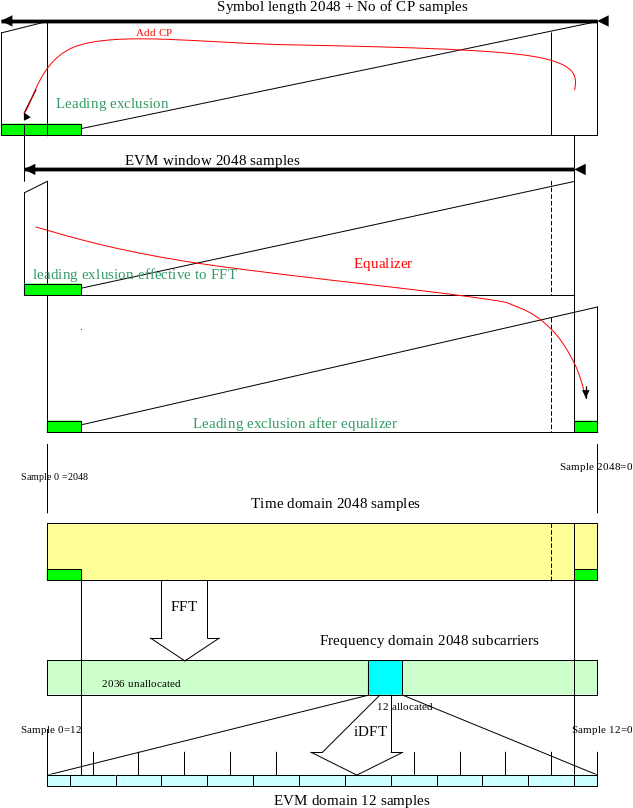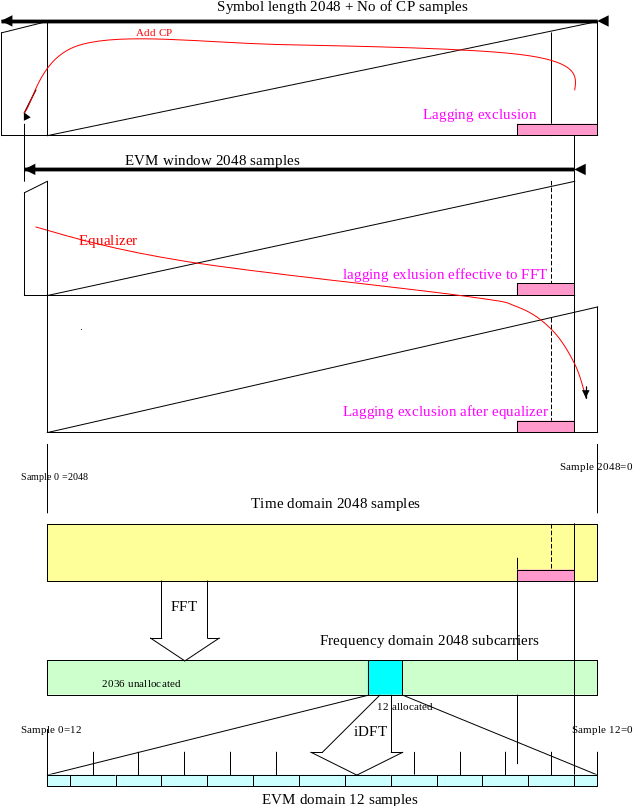## E.7 EVM with exclusion period

36.521-13GPPEvolved Universal Terrestrial Radio Access (E-UTRA)Part 1: Conformance testingRadio transmission and receptionRelease 17TSUser Equipment (UE) conformance specification

## E.7.1 General

EVM with exclusion periods is defined in clause 6.5.2.1.1, third paragraph. For PUCCH entire symbols are excluded, if applicable. For PUSCH fractions of symbols are excluded, if applicable. The exclusion period for PUSCH is defined at the air interface, leading to exclusion periods in the EVM domain. The necessary mapping is described in this clause.

## E.7.2 The model

The exclusion period in the time domain has corresponding periods in the quasi time domains (Table E.7.2). The mapping of corresponding periods needs only scaling and cyclic shifting.

The algorithm below uses a sampling frequency 30.72 MHz and FFT-width 2048 for all bandwidths. Bandwidth-adapted sampling frequencies and FFT-widths are not excluded. Only normal cyclic prefix is mentioned in the model without excluding the extended CP.

Table. E.7.2: Model for mapping exclusion period in the time domain

 TX Channel EVM meter Operation DFT iFFT FFT iDFT Meaning Modulation symbols Precoded symbols BB samples BB samples Precoded symbols demodula-ted symbols No of samples allocated Sub Carriers allocated subcarriers + unallocated subcarriers = 2048 allocated subcarriers + unallocated subcarriers + CP samples 2048, position depending on EVM window allocated subcarriers allocated subcarriers Domain Quasi time domain Frequency domain Time domain Time domain Frequency domain Quasi time domain text below 1 2 3 4 7 7 8 9 11

1. A sequence of complex valued modulation symbols are Transform-Precoded (DFT) according to 36.211 clause 5.3.3. The size of this transformation is the number of allocated subcarriers.

2. The outcome of (1) is supplemented by 0 for the non allocated subcarriers. In sum 2048 subcarriers.

3. The baseband time signal (without CP) is then calculated by a iFFT according to 36.211 clause 5.6

4. (3) is then supplemented by a cyclic prefix (144 or 160 samples) leading to 2192 or 2208 samples. (144 CP samples = 144 tail samples from the data field)

5. (4) is transmitted over the channel and sampled by the EVM meter.

6. In case of an exclusion period those samples of (5) are marked, where the exclusion applies. The exclusion period is an unbroken leading or lagging exclusion period next to a subframe or timeslot boarder.

7.Depending on early or late EVM-window a subset of 2048 samples (out of 2192 or 2208 samples) are the input for the subsequent FFT. These samples may or may not comprise marked samples. The result are 2048 frequency domain samples.

8. The non allocated subcarriers are removed from the 2048 samples.

9. (8) is then iDFT transformed. The result are demodulated complex valued symbols in the same domain as (1)

10. Step 7, 8 and 9 are modified by an equalizer algorithm.
For the purpose of this clause, the equalizer partly re-does step 4 (CP insertion):
The equalizer algorithm cuts that subset of CP samples, covered by the FFT, from the head and copies it to the tail of the data field.

11. The result of (10) is: complex valued symbols in the same sequence as in (1) They are compared with (1) symbol by symbol for EVM. Due to exclusion in the time domain (6) we have marked corresponding symbols, which are disregarded for EVM.

12. From step 1 to 4 the number of samples is expanded. A subset of expanded samples is marked as excluded. Form step 6 to step 9 the number of samples is compressed, leading to a non integer number of samples, marked as excluded. The number of marked samples in this domain is rounded up at the expense of the EVM samples

## E.7.3 Illustration

The figures below illustrate the cyclic shift due to the equalizer and scaling.Figure E.7.3-1: leading exclusion period (when number of RBs=1)Figure E.7.3-2: lagging exclusion period (when number of RBs=1)

Legend to figure E.7.3-1

The figure contains 6 related subfigures.

The upper 3 triangles illustrate the cyclic shift due to EVM windowing and equalizer.

The lower 3 boxes illustrate the mapping from the time domain, where the exclusion period is defined, into the EVM domain, where EVM samples are actually excluded.

Cyclic shift

The leading exclusion period covers the entire CP and a part of the data field. The EVM window masks a part of the exclusion period. For the purpose of this annex, the equalizer re-arranges the time domain samples into the original order, splitting the exclusion period into two parts.

Mapping

The split exclusion period (after cyclic shift) is copied from above. The frequency domain is derived by Fast Fourier Transformation from the time domain and represent the frequency domain with 2048 subcarriers, 12 of them are allocated, and the rest is unallocated. The 12 allocated subcarriers are iDFT transformed into the EVM domain comprising 12 samples. Note that all 3 domains are displayed cyclically: the leftmost sample is identical to the rightmost sample.

The two transformations map the time domain into the EVM domain, carrying out a compression of samples 12/2048. In spite of the compression, there is a correspondence of ranges in the time domain and in the EVM domain. One sample in the EVM domain comprises a range, which is influenced from (and only from) the equivalent samples in the time domain vertically above. Note that this correspondence holds irrespective of the position of the 12 allocated samples in the frequency domain.

 Sample No in the EVM domain Influence(exclusion) from the time domain EVM exclusion 12=0 full excluded 1 partly excluded 2 to 11 none counted

Example lagging exclusion example (figure E.7.3-2)

 Sample No in the EVM domain Influence(exclusion) from the time domain EVM exclusion 12=0 none counted 1 to 9 none counted 10 partly excluded 11 full excluded

## E.7.4 Formula

The exclusion period is defined in µs at the air interface.

Convert the µs’s into No of samples in the time domain.

No of exclusion samples (before EVM windowing and equalizer) is calculated from:

No of exclusion sample = ceil (30.72 * Exclusion μs)

then, EVM windowing and equalizer is applied by 2048 samples based cyclic shift process.

(the upper 3 triangles illustrate in fig. E.7.3-1 and -2.)

Determine the indices k, to be excluded in the time domain, according to fig. E.7.3-1 and -2

(after application of EVM windowing and equaliser, original sample order,

k = subset from the set (0 to 2047) )

The indices l in the EVM domain, to be excluded, are:

l = [ round (k*12* LCRBs / 2048)]mod(12*LCRBs)

with LCRBs number of allocated resource blocks